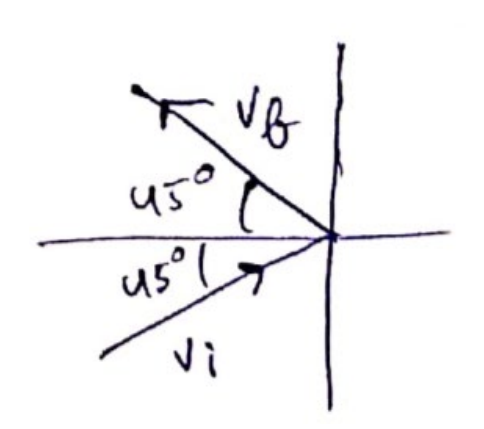# Solve the following :Question:

A ball of mass $50 \mathrm{~g}$ moving at a speed of $2.0 \mathrm{~m} / \mathrm{s}$ strikes a plane surface at an angle of incidence $45^{\circ}$. The ball is reflected by the plane at equal angle of reflection with the same speed. Calculate (a) the magnitude of the change in momentum of the ball. (b) thechange in the magnitude of the momentum of the ball.

Solution:$V_{i}=+v \cos 45^{\circ} \hat{\imath}+v \sin 45^{\circ} \hat{\jmath}$

$V_{f}=-v \cos 45^{\circ} \hat{\imath}+v \sin 45^{\circ} \hat{\jmath}$

(a) change in momentum

$m\left(v_{i}-v_{f}\right)=m .2 v \cos 45^{\circ} \hat{\imath}$

$\Delta \vec{p}=2 \times 0.05 \times 2 \frac{1}{\sqrt{2}} \hat{\imath}$

$\Delta \vec{p}=\frac{0.2}{\sqrt{2}} \hat{\imath}$

$|\Delta p|=\frac{}{\sqrt{2}}=\frac{}{10}=0.14$

(b) change in momentum magnitude

$m\left(\left|\overrightarrow{v_{i}}\right|-\left|\overrightarrow{v_{f}}\right|\right)=m(v-v)=0$